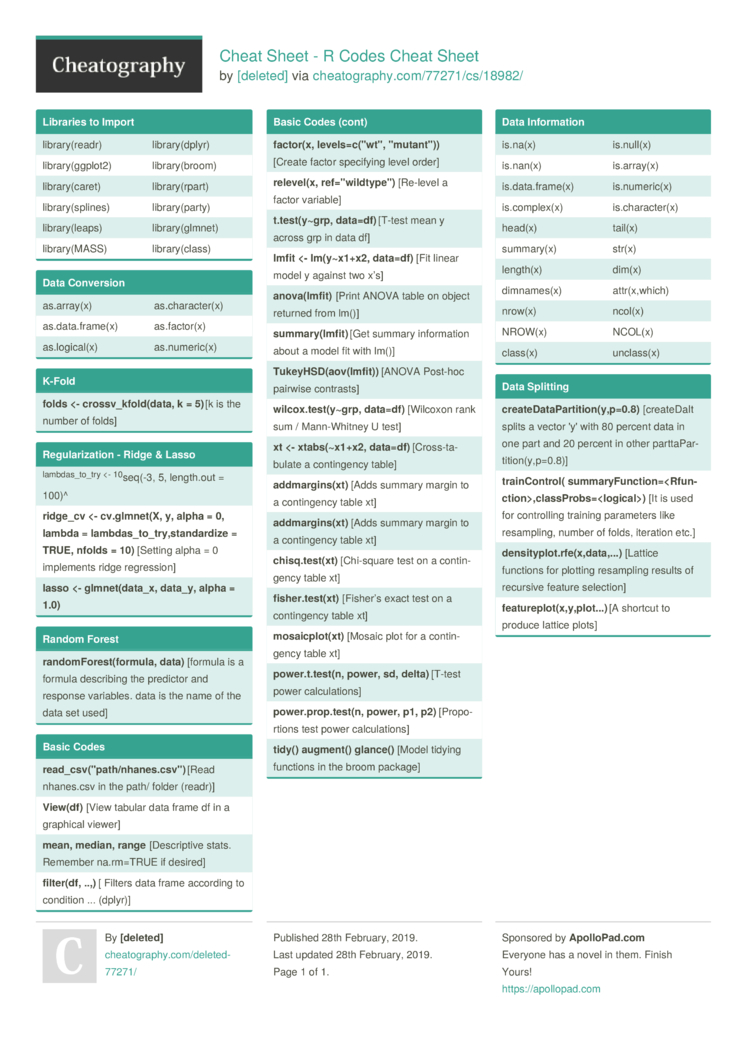Cheat Sheet - R Codes Cheat Sheet by [deleted]

Libraries to Import

 librar­y(r­eadr) librar­y(d­plyr) librar­y(g­gplot2) librar­y(b­room) librar­y(c­aret) librar­y(r­part) librar­y(s­plines) librar­y(p­arty) librar­y(l­eaps) librar­y(g­lmnet) librar­y(MASS) librar­y(c­lass)

Data Conversion

 as.arr­ay(x) as.cha­rac­ter(x) as.dat­a.f­rame(x) as.fac­tor(x) as.log­ical(x) as.num­eric(x)

K-Fold

 folds <- crossv­_kf­old­(data, k = 5) [k is the number of folds]

Regula­riz­ation - Ridge & Lasso

 lambd­as_­to_try <- 10seq(-3, 5, length.out = 100)^ ridge_cv <- cv.glm­net(X, y, alpha = 0, lambda = lambda­s_t­o_t­ry,­sta­nda­rdize = TRUE, nfolds = 10) [Setting alpha = 0 implements ridge regres­sion] lasso <- glmnet­(da­ta_x, data_y, alpha = 1.0)

Random Forest

 rand­omF­ore­st(­for­mula, data) [formula is a formula describing the predictor and response variables. data is the name of the data set used]

Basic Codes

 read­_cs­v("p­ath­/nh­ane­s.c­sv") [Read nhanes.csv in the path/ folder (readr)] View­(df) [View tabular data frame df in a graphical viewer] mean, median, range [Descr­iptive stats. Remember na.rm=TRUE if desired] filt­er(df, ..,) [ Filters data frame according to condition ... (dplyr)] fact­or(x, levels­=c(­"­wt", "­mut­ant­")) [Create factor specifying level order] rele­vel(x, ref="wi­ldt­ype­") [Re-level a factor variable] t.te­st(­y~grp, data=df) [T-test mean y across grp in data df] lmfit <- lm(y~x­1+x2, data=df) [Fit linear model y against two x’s] anov­a(l­mfit) [Print ANOVA table on object returned from lm()] summ­ary­(lm­fit) [Get summary inform­ation about a model fit with lm()] Tuke­yHS­D(a­ov(­lmf­it)) [ANOVA Post-hoc pairwise contrasts] wilc­ox.t­es­t(y­~grp, data=df) [Wilcoxon rank sum / Mann-W­hitney U test] xt <- xtabs(­~x1+x2, data=df) [Cross­-ta­bulate a contin­gency table] addm­arg­ins­(xt) [Adds summary margin to a contin­gency table xt] addm­arg­ins­(xt) [Adds summary margin to a contin­gency table xt] chis­q.t­est­(xt) [Chi-s­quare test on a contin­gency table xt] fish­er.t­es­t(xt) [Fisher’s exact test on a contin­gency table xt] mosa­icp­lot­(xt) [Mosaic plot for a contin­gency table xt] powe­r.t.te­st(n, power, sd, delta) [T-test power calcul­ations] powe­r.p­rop.te­st(n, power, p1, p2) [Propo­rtions test power calcul­ations] tidy() augment() glance() [Model tidying functions in the broom package]

Data Inform­ation

 is.na(x) is.null(x) is.nan(x) is.arr­ay(x) is.dat­a.f­rame(x) is.num­eric(x) is.com­plex(x) is.cha­rac­ter(x) head(x) tail(x) summary(x) str(x) length(x) dim(x) dimnam­es(x) attr(x­,which) nrow(x) ncol(x) NROW(x) NCOL(x) class(x) unclass(x)

Data Splitting

 crea­teD­ata­Par­tit­ion­(y,­p=0.8) [creat­eDaIt splits a vector 'y' with 80 percent data in one part and 20 percent in other partta­Par­tit­ion­(y,­p=0.8)] trai­nCo­ntrol( summar­yFu­nct­ion­=­,cl­ass­Pro­bs=­) [It is used for contro­lling training parameters like resamp­ling, number of folds, iteration etc.] dens­ity­plo­t.r­fe(­x,d­ata­,...) [Lattice functions for plotting resampling results of recursive feature selection] feat­ure­plo­t(x­,y,­plo­t...) [A shortcut to produce lattice plots]

Download the Cheat Sheet - R Codes Cheat Sheet1 Page
//media.cheatography.com/storage/thumb/deleted-77271_cheat-sheet-r-codes.750.jpg

PDF (recommended)